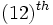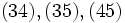# Semidirect product is not left-cancellative for finite groups

## Statement

### Statement from the external semidirect product viewpoint

It is possible to have finite groups$A,B,C$, and semidirect products, such that:$A \rtimes B \cong A \rtimes C$,

but$B$ is not isomorphic to$C$.

### Statement from the internal semidirect product viewpoint

We can construct a finite group$P$ with subgroups$H,K,L,M$ such that:$P = H \rtimes L = K \rtimes M$,

where$H$ is isomorphic to$K$ but$L$ is not isomorphic to$M$.

## Proof

### Example involving the upper triangular matrices

Suppose$p$ is any prime, and let$G := U(5,p)$ be the group of upper-triangular unipotent$5 \times 5$ matrices over the field of$p$ elements. Let$P$ be the subgroup of$G$ comprising those matrices where the$(12)^{th}$ entry is zero. Then,$P$ is a group of order$p^9$.

By fact (1), we have that$G$ has two subgroups that are Abelian of maximum order: the top right rectangle groups of dimensions$2 \times 3$ and$3 \times 2$ respectively. Call these subgroups$H$ and$K$ respectively. Then, observe that:

• Both$H$ and$K$ are also Abelian subgroups of maximum order in$P$. Moreover, they are the only Abelian subgroups of maximum order in$P$ since they are the only Abelian subgroups of maximum order in$G$.
•$H$ and$K$ are isomorphic -- in fact, they are conjugate subgroups inside the bigger group$GL(5,p)$. This conjugation restricts to an automorphism of$G$, but not of$P$.
• Both$H$ and$K$ are normal in$G$, and hence in$P$.
• The subgroup with nonzero entries in the$(34),(35),(45)$ positions is a permutable complement to$H$ in$P$. Call this subgroup$L$. Then$P$ is an internal semidirect product of$H$ and$L$.$L$ is isomorphic to the prime-cube order group:U3p.
• The subgroup with nonzero entries in the$(13),(23),(45)$ positions is a permutable complement to$K$ in$G$. Call this subgroup$M$. Then,$P$ is an internal semidirect product of$K$ and$M$. Note that$M$ is isomorphic to the elementary Abelian group of order$p^3$.
• Thus, we have$P = H \rtimes L = K \rtimes M$, with$H \cong K$ but$L$ not isomorphic to$M$.